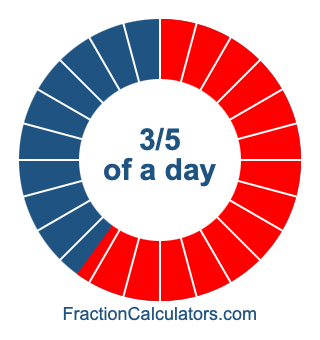What is 3/5 of a day?Here we will show you how to calculate exactly how many hours, minutes, and seconds there are in 3/5 of a day. We will also illustrate what 3/5 of a day looks like on a pie chart.

Step 1) Calculate hours
We start by calculating the total number of whole hours in 3/5 of a day. There are 24 hours in a day. Therefore, we multiply 3/5 by 24. The whole number of the product we get is the number of hours.

3/5 × 24 = 72/5
72/5 = 14 2/5
= 14 hours

Step 2) Calculate minutes
Next we calculate the minutes. There are 60 minutes per hour. Therefore, we multiply the leftover fraction part from Step 1 by 60. The whole number of the product we get is the number of minutes.

2/5 × 60 = 120/5
120/5 = 24
= 24 minutes

Step 3) Calculate seconds
Finally, we calculate the seconds. There are 60 seconds per minute. Therefore, we multiply the leftover fraction part from Step 2 by 60. The product we get is the number of seconds.

0 × 60 = 0
0 = 0
= 0 seconds

Now we have the hours, minutes and seconds of 3/5 of day. When we put it all together we get our answer as follows:

3/5 of a day = 14 hours & 24 minutes

Below is a pie chart showing 3/5 of day. To create the image, we first colored the whole pie in blue, and then we covered 3/5 of it in red.Note that each pie slice above is one hour, and the whole pie is one day. Therefore, there are twenty-four slices.

Fraction of a Day Calculator
Here you can calculate another fraction of a day.

What is  /  of a day?

What is 3/6 of a day?
Here is the next fraction of a day on our list that we have calculated and illustrated for you.